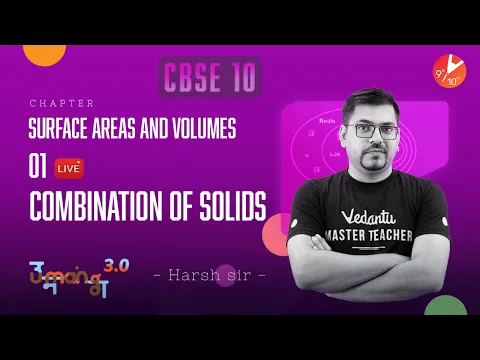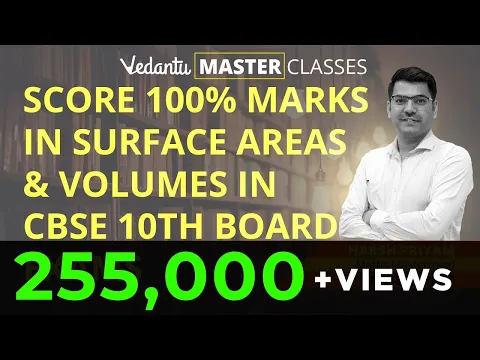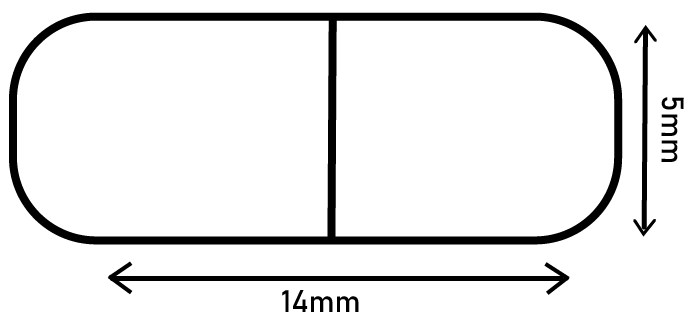Courses
Courses for Kids
Free study material
Offline Centres
More

# NCERT Solutions for Class 10 Maths Chapter 13 - ExerciseLast updated date: 05th Dec 2023
Total views: 533.4k
Views today: 12.33k

## NCERT Solutions for Class 10 Maths Chapter 13 Surface Areas and Volumes (Ex 13.1) Exercise 13.1

Free PDF download of NCERT Solutions for Class 10 Maths Chapter 13 Exercise 13.1 (Ex 13.1) and all chapter exercises at one place prepared by an expert teacher as per NCERT (CBSE) books guidelines. Class 10 Maths Chapter 13 Surface Areas And Volumes Exercise 13.1 Questions with Solutions to help you to revise complete Syllabus and Score More marks. Register and get all exercise solutions in your emails. CBSE Solutions for all classes and subjects are free.

You can also Download NCERT Solutions Class 10 Maths to help you to revise the complete Syllabus and score more marks in your examinations. Along with Maths, you will also find NCERT Solutions for Class 10 Science on Vedantu.

NCERT Class 10 Maths Chapter 13 is on the surface areas and volumes. This chapter comprises 6 major parts that help to understand the fundamentals of surface areas and volumes properly. The following is a list of the 6 important topics that are covered in Class 10 Chapter 13 Surface Areas and Volumes.

 Sl. No. Topics 1 An Introduction 2 Surface Area of a Combination of Solids 3 Volume of a Combination of Solids 4 Conversion of Solid From One Shape to Another 5 Frustum of a Cone 6 A Summary

We advise students to go through these individual topics carefully to get a precise understanding of the chapter.

### Importance of Learning About Surface Areas and Volumes

Measurements are an important part of mathematics and measuring surface areas and volumes helps greatly in the construction and determination of dimensions of any given object. Surface area is the two-dimensional area bounded by lengths, widths, and edges, and volume is the amount of space contained in any given three-dimensional object. Solving and practising the sums given in this chapter will enable students to compute surface areas and volumes of various figures easily in exams as well as in real life.

Watch videos on
NCERT Solutions for Class 10 Maths Chapter 13 - ExerciseSurface Areas and Volumes Full Chapter in One Shot | CBSE Class 10 Maths Chap 13 | Term 2 Solutions
Vedantu 9&10
SubscribeShare
8.2K likes
130.3K Views
1 year agoSurface Areas and Volumes L-1 [ Combination of Solids ] CBSE Class 10 Maths Ch13 | Term 2 Solutions
Vedantu 9&10
6K likes
155.5K Views
1 year ago
Play QuizSurface Area and Volume L1 | Surface Areas and Volumes of Combinations of Solids CBSE Class 10 Maths
Vedantu 9&10
6.9K likes
168.5K Views
3 years ago
Play QuizMaster Surface Area and Volume Class 10 | Surface Area and Volume All Formulas | Frustum of a Cone
Vedantu 9&10
6.8K likes
274.7K Views
5 years ago

## Access NCERT Solutions for Class 10 Maths Chapter 13 – Surface Areas and Volumes

### Exercise 13.1

1. Two cubes each of volume $64\,\,c{{m}^{3}}$ are joined end to end. Find the surface area of the resulting cuboids.

Ans: Given: Volume of cubes $=\text{ }64\text{ }c{{m}^{3}}$

${{\left( Edge \right)}^{3}}=\text{ }64$

Edge $=\text{ }4\text{ }cm$

(Image Will Be Updated Soon)

If cubes are joined end to end, the dimensions of the resulting cuboid will be $4\text{ }cm,4cm,8\text{ }cm$.

Surface area of cuboids:

$\Rightarrow 2\left( lb+bh+lh \right)$

$\Rightarrow 2\left( \left( 4 \right)\left( 4 \right)+\left( 4 \right)\left( 8 \right)+\left( 4 \right)\left( 8 \right) \right)$

$\Rightarrow 2\left( 16+32+32 \right)$

$\Rightarrow 2\left( 16+64 \right)$

$\Rightarrow 2\left( 80 \right)=160\,c{{m}^{2}}$

2. A vessel is in the form of a hollow hemisphere mounted by a hollow cylinder. The diameter of the hemisphere is $14$cm and the total height of the vessel is $13$cm. Find the inner surface area of the vessel. $\left[ Use\,\,\pi =\dfrac{22}{7} \right]$.

Ans:

(Image Will Be Updated Soon)

It can be observed that the radius $\left( r \right)$of the cylindrical part and the hemispherical part is the same (i.e.,$7\text{ }cm$).

Height of hemispherical part $=Radius=7cm$

Height of cylindrical part $\left( h \right)=13-7=6\text{ }cm$

Inner surface area of the vessel : $\Rightarrow CSA\text{ }of\text{ }cylindrical\text{ }part+CSA\text{ }of\text{ }hemispherical\text{ }part$

$\Rightarrow 2\pi rh+2\pi {{r}^{2}}$

$\Rightarrow \left[ 2\left( \dfrac{22}{7} \right)\left( 7 \right)\left( 6 \right) \right]+\left[ 2\left( \dfrac{22}{7} \right)\left( 7 \right)\left( 7 \right) \right]$

$\Rightarrow 44\left( 6+7 \right)$

$\Rightarrow 44\times 13=572\,c{{m}^{2}}$

3. A toy is in the form of a cone of radius $3.5cm$ mounted on a hemisphere of same radius. The total height of the toy is $15.5\,cm$. Find the total surface area of the toy. $\left[ Use\,\,\pi =\dfrac{22}{7} \right]$

Ans:

(Image Will Be Updated Soon)

It can be observed that the radius of the conical part and the hemispherical part is the same (i.e., 3.5 cm).

Height of hemispherical part $=Radius\left( r \right)=3.5=\dfrac{7}{2}\text{ }cm$

Height of conical part $\left( h \right)=5.5-3.5=12\text{ }cm$

Slant height (l) of conical part:

$\Rightarrow \sqrt{{{r}^{2}}+{{h}^{2}}}$

$\Rightarrow \sqrt{{{\left( \dfrac{7}{2} \right)}^{2}}+{{\left( 12 \right)}^{2}}}=\sqrt{\dfrac{49}{4}+144}$

$\Rightarrow \sqrt{\dfrac{625}{4}}=\dfrac{25}{2}$

Total surface area of toy:

$\Rightarrow CSA\text{ }of\text{ }conical\text{ }part+CSA\text{ }of\text{ }hemispherical\text{ }part$

$\Rightarrow \pi rl+2\pi {{r}^{2}}$

$\Rightarrow \left[ \left( \frac{22}{7} \right)\left( \frac{7}{2} \right)\left( \frac{25}{2} \right) \right]+\left[ 2{{\left( \frac{7}{2} \right)}^{2}}\frac{22}{7} \right]$

$\Rightarrow 137.5+77=214.5\,c{{m}^{2}}$

4. A cubical block of side $7\,cm$ is surmounted by a hemisphere. What is the greatest diameter the hemisphere can have? Find the surface area of the solid. $\left[ Use\,\,\pi =\dfrac{22}{7} \right]$

Ans:

(Image Will Be Updated Soon)

From the figure, it can be observed that the greatest diameter possible for such a hemisphere is equal to the cube’s edge, i.e.,$7cm$.

Radius (r) of hemispherical part$=\dfrac{7}{2}=3.5cm$

Total surface area of solid:

Surface area of cubical part + CSA of hemispherical = Area of base of hemispherical part

$\Rightarrow 6{{\left( edge \right)}^{2}}+2\pi {{r}^{2}}-\pi {{r}^{2}}$

$\Rightarrow 6{{\left( edge \right)}^{2}}+\pi {{r}^{2}}$

$\Rightarrow 6{{\left( 7 \right)}^{2}}+\left( \dfrac{22}{7} \right){{\left( \dfrac{7}{2} \right)}^{2}}$

$\Rightarrow 294+38.5=332.5\,c{{m}^{2}}$

5. A hemispherical depression is cut out from one face of a cubical wooden block such that the diameter $l$ of the hemisphere is equal to the edge of the cube. Determine the surface area of the remaining solid.

Ans:

(Image Will Be Updated Soon)

Diameter of hemisphere $=Edge\text{ }of\text{ }cube=L$

Radius of hemisphere $=\dfrac{L}{2}$

Total surface area of solid:

Surface area of cubical part + CSA of hemispherical part = Area of base of hemispherical part

$\Rightarrow 6{{\left( edge \right)}^{2}}+2\pi {{r}^{2}}-\pi {{r}^{2}}$

$\Rightarrow 6{{\left( edge \right)}^{2}}+\pi {{r}^{2}}$

$\Rightarrow 6\left( {{L}^{2}} \right)+\frac{\pi {{L}^{2}}}{4}$

$\Rightarrow 6{{L}^{2}}+\dfrac{\pi {{L}^{2}}}{4}$

$=\dfrac{1}{4}\left( 24+\pi \right){{L}^{2}}$

6. A medicine capsule is in the shape of a cylinder with two hemispheres stuck to each of its end (see the given figure). The length of the entire capsule is $14$ mm and the diameter of the capsule is 5 mm. Find its surface area [use $\pi =\frac{22}{7}$]Ans: It can be observed that,

$=\dfrac{Diameter\,of\,the\,capsule}{2}$

$=\dfrac{5}{2}$

Length of  cylindrical part ( h )=Length of the entire capsule$-2\times r$

$=14-5$

$=9\,mm$

Surface area of capsule:

2CSA of hemispherical part+CSA of cylindrical part

$\Rightarrow 2\left( 2\pi {{r}^{2}} \right)+2\pi rh$

$\Rightarrow 4\pi {{\left( \dfrac{5}{2} \right)}^{2}}+2\pi \left( \dfrac{5}{2} \right)\left( 9 \right)$

$\Rightarrow 25\pi +45\pi$

$\Rightarrow 70\left( \dfrac{22}{7} \right)$

$\Rightarrow 220\,\,m{{m}^{2}}$

7. A tent is in the shape of a cylinder surmounted by a conical top. If the height and diameter of the cylindrical part are $\mathbf{2}.\mathbf{1}\text{ }\mathbf{m}$and $\mathbf{4}\text{ }\mathbf{m}$ respectively, and the slant height of the top is$\mathbf{2}.\mathbf{8}\text{ }\mathbf{m}$, find the area of the canvas used for making the tent. Also, find the cost of the canvas of the tent at the rate of $\mathbf{Rs}\text{ }\mathbf{500}\text{ }\mathbf{per}\text{ }{{\mathbf{m}}^{2}}$. (Note that the base of the tent will not be covered with canvas.) $\left[ Use\,\,\pi =\dfrac{22}{7} \right]$

Ans:

(Image Will Be Updated Soon)

Given that,

Height (h) of the cylindrical part $=2.1m$

Diameter of the cylindrical part $=4m$

Radius of the cylindrical part $=2m$

Slant height (l) of conical part $=2.8m$

Area of canvas used:

CSA of conical part+CSA of cylindrical part

$\Rightarrow \pi rl+2\pi rh$

$\Rightarrow \pi \left( 2 \right)\left( 2.8 \right)+2\pi \left( 2 \right)\left( 2.1 \right)$

$\Rightarrow 2\pi \left[ 2.8+2\left( 2.1 \right) \right]$

$\Rightarrow 2\pi \left[ 2.8+4.2 \right]=2\left( \dfrac{22}{7} \right)\left( 7 \right)$

$\Rightarrow 44{{m}^{2}}$

Cost of $1\text{ }{{m}^{2}}$canvas = $Rs\text{ }500$

Cost of $44\text{ }{{m}^{2}}$canvas $=44\times 500=22000$

Therefore, it will cost $Rs\text{ }22000$for making such a tent.

8. From a solid cylinder whose height is $\mathbf{2}.\mathbf{4}\text{ }\mathbf{cm}$ and diameter $\mathbf{1}.\mathbf{4}\text{ }\mathbf{cm}$, a conical cavity of the same height and same diameter is hollowed out. Find the total surface area of the remaining solid to the nearest $\mathbf{c}{{\mathbf{m}}^{2}}$. [ Use $\pi =\frac{22}{7}$ ]

Ans:

(Image Will Be Updated Soon)

Given:

Height (h) of the conical part=Height (h) of the cylindrical part$=2.4\text{ }cm$

Diameter of the cylindrical part = 1.4 cm

Therefore, radius (r) of the cylindrical part $=0.7cm$

Slant height (l) of conical part:

$\Rightarrow \sqrt{{{r}^{2}}+{{h}^{2}}}$

$\Rightarrow \sqrt{{{\left( 0.7 \right)}^{2}}+{{\left( 2.4 \right)}^{2}}}$

$\Rightarrow \sqrt{0.49+5.76}$

$\Rightarrow \sqrt{0.49+5.76}$

$\Rightarrow \sqrt{6.25}=2.5$

Total surface area of the remaining solid will be:

CSA of cylindrical part+CSA of conical part +Area of cylindrical base \Rightarrow 2\pi rh+\pi rl+\pi {{r}^{2}}\Rightarrow \left( 2\times \dfrac{22}{7}\times 0.7\times 2.4 \right)+\left( \dfrac{22}{7}\times 0.7\times 2.5 \right)+\left( \dfrac{22}{7}\times 0.7\times 0.7 \right)\Rightarrow \left( 4.4\times 2.4 \right)+\left( 2.2\times 2.5 \right)+\left( 2.2\times 0.7 \right)\Rightarrow 10.56+5.50+1.54=17.60~\text{c}{{\text{m}}^{2}}$The total surface area of the remaining solid to the nearest$\text{c}{{\text{m}}^{2}}$is$18~\text{c}{{\text{m}}^{2}}$9. A wooden article was made by scooping out a hemisphere from each end of a solid cylinder, as shown in the given figure. If the height of the cylinder is $\mathbf{10}\text{ }\mathbf{cm}$, and its base is of radius $\mathbf{3}.\mathbf{5}\text{ }\mathbf{cm}$, find the total surface area of the article.$\left[ Use\,\,\pi =\dfrac{22}{7} \right]$Ans: (Image Will Be Updated Soon) Given: Radius (r) of cylindrical part =Radius( r) of hemispherical part=3.5 cm Height of cylindrical part (h) $=10\text{ }cm$ Surface area of article: CSA of cylindrical part+2 CSA of hemispherical part$\Rightarrow 2\pi rh+2\left( 2\pi {{r}^{2}} \right)\Rightarrow 2\pi \left( 3.5 \right)\left( 10 \right)+2\left( 2\pi  \right){{\left( 3.5 \right)}^{2}}\Rightarrow 70\pi +49\pi \Rightarrow 119\pi \Rightarrow 17\left( 22 \right)=374\,c{{m}^{2}}\$

### NCERT Solutions for Class 10 Maths Chapter 13 Surface Areas and Volumes Exercise 13.1

Opting for the NCERT solutions for Ex 13.1 Class 10 Maths is considered as the best option for the CBSE students when it comes to exam preparation. This chapter consists of many exercises. Out of which we have provided the Exercise 13.1 Class 10 Maths NCERT solutions on this page in PDF format. You can download this solution as per your convenience or you can study it directly from our website/ app online.

Vedantu in-house subject matter experts have solved the problems/ questions from the exercise with the utmost care and by following all the guidelines by CBSE. Class 10 students who are thorough with all the concepts from the Subject Surface Areas and Volumes textbook and quite well-versed with all the problems from the exercises given in it, then any student can easily score the highest possible marks in the final exam. With the help of this Class 10 Maths Chapter 13 Exercise 13.1 solutions, students can easily understand the pattern of questions that can be asked in the exam from this chapter and also learn the marks weightage of the chapter. So that they can prepare themselves accordingly for the final exam.

Besides these NCERT solutions for Class 10 Maths Chapter 13 Exercise 13.1, there are plenty of exercises in this chapter which contain innumerable questions as well. All these questions are solved/answered by our in-house subject experts as mentioned earlier. Hence all of these are bound to be of superior quality and anyone can refer to these during the time of exam preparation. In order to score the best possible marks in the class, it is really important to understand all the concepts of the textbooks and solve the problems from the exercises given next to it.

Do not delay any more. Download the NCERT solutions for Class 10 Maths Chapter 13 Exercise 13.1 from Vedantu website now for better exam preparation. If you have the Vedantu app in your phone, you can download the same through the app as well. The best part of these solutions is these can be accessed both online and offline as well.

### NCERT Solutions for Class 10 Maths Chapter 13 Exercises

 Chapter 13 Surface Areas and Volumes All Exercises in PDF Format Exercise 13.2 8 Questions and Solutions Exercise 13.3 9 Questions and Solutions Exercise 13.4 5 Questions and Solutions Exercise 13.5 7 Questions and Solutions

## FAQs on NCERT Solutions for Class 10 Maths Chapter 13 - Exercise

1. What are the topics and sub-topics of the Class 10 Maths Chapter 13?

A. There are quite a few topics and sub-topics of this chapter. They are- 1) Surface Areas and Volumes, 2) Introduction, 3) Surface Area of a Combination Of Solid, 4) Volume of a combination of Solids, 5) Conversion of Solid From One Shape to Another, 6) Frustum of a Cone.

2. How many questions are there in Class 10 Maths Chapter 13 Exercise 13.1 of the NCERT textbook?

A. Chapter 13 Exercise 13.1 of Class 10 Mathematics NCERT book contains a total of seven long and two short questions. We, at Vedantu, provide the answers for the same as well in our solutions PDF, which you will find on our website and mobile application. You can download these solutions without spending a penny and use them for better exam preparation.

3. What is a frustum of a cone?

A. Here goes the definition- when we cut through a cone or cone-shaped object with a plane parallel to the base and remove the cone that is formed on one side of the plane, now the part which is now leftover on the other side of the plane is called as a frustum of a cone.

4. How is Vedantu’s NCERT Solutions for Class 10 Maths Chapter 13 Exercise 13.1 prepared?

A. Class 10 students who are looking for a better NCERT Solutions for Mathematics should definitely look into the solutions PDF provided by Vedantu, India’s no.1 ed-tech company. All the answers provided in these solutions are 100% accurate. The problems on this exercise have been answered by our Maths expertise, to make students understand the theories in a better way. Topics covered in these materials are according to NCERT syllabus and guidelines. Therefore, solutions for Maths class 10, are very helpful for students who are doing the preparation for the exams and to score good marks.